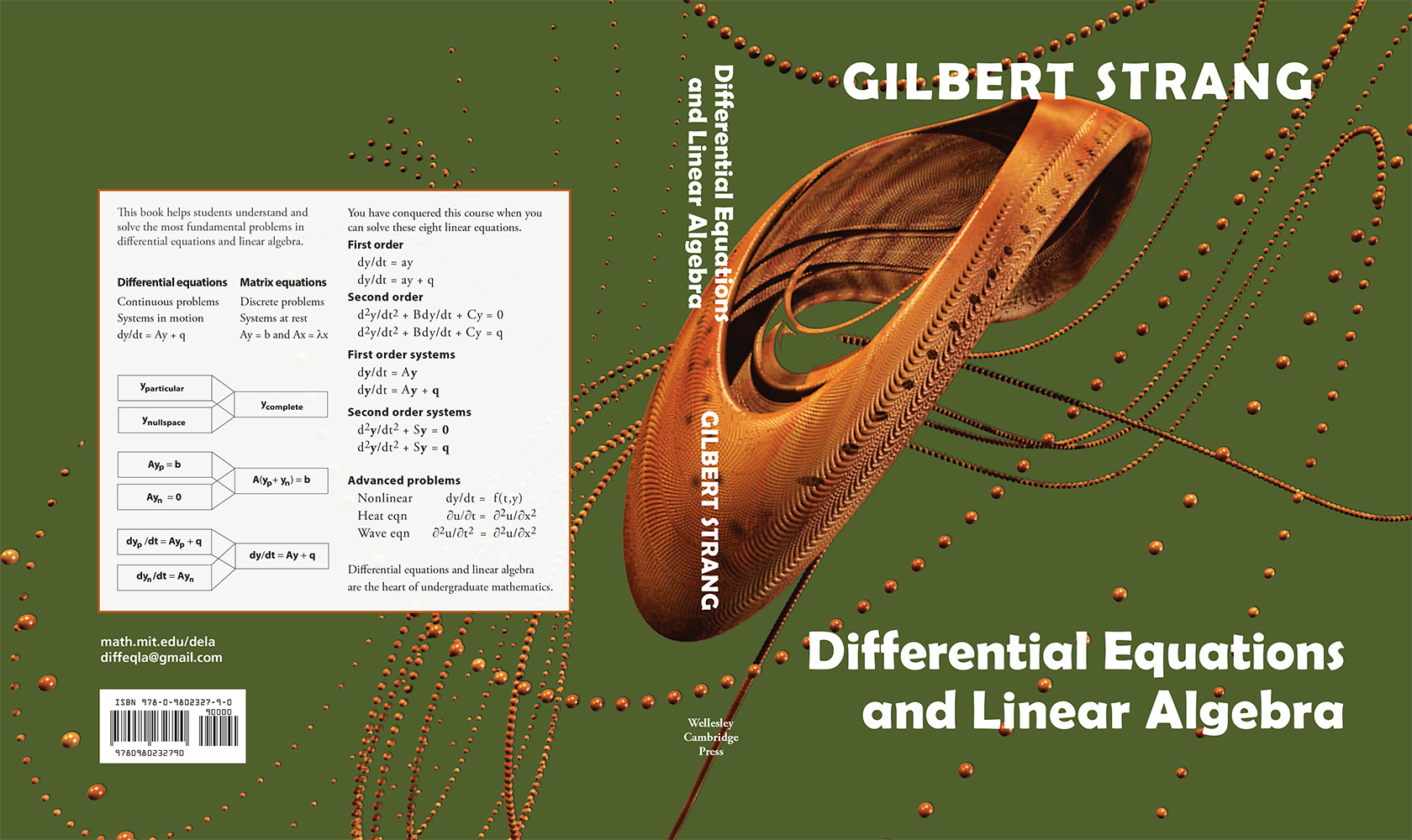# Welcome!

## Book Order Form

For orders and requests, email diffeqla@gmail.com

55 short videos have been created to present the
main ideas for differential equations in an active way.
Cleve Moler (who created MATLAB) developed a parallel series
of videos about numerical solutions that presents increasingly
accurate and professional codes from MATLAB's ODE Suite.

Here is the link to the Strang and Moler series on MIT OpenCoureWare.
http://ocw.mit.edu/resources/res-18-009-learn-differential-equations-up-close-with-gilbert-strang-and-cleve-moler-fall-2015/

See the MIT site for links on YouTube, iTunes and Internet Archive.

Linear algebra videos by Professor Strang are already on YouTube and OpenCourseWare ocw.mit.edu (Math 18.06 and 18.06 SC)
Here are the full links to those:
18.06 - http://ocw.mit.edu/courses/mathematics/18-06-linear-algebra-spring-2010/
18.06 SC - http://ocw.mit.edu/courses/mathematics/18-06sc-linear-algebra-fall-2011/

#### Samples of the book

Chapter 1: First Order Equations
1.1 Four Examples : Linear versus Nonlinear
Chapter 2: Second Order Equations
2.1 Second Derivatives in Science and Engineering
Chapter 3: Graphical and Numerical Methods
3.2 Sources, Sinks, Saddles, and Spirals
Chapter 4: Linear Equations and Inverse Matrices
4.1 Two Pictures of Linear Equations
Chapter 5: Vector Spaces and Subspaces
5.1 The Column Space of a Matrix
Chapter 6: Eigenvalues and Eigenvectors
6.1 Introduction to Eigenvalues
Chapter 7: Applied Mathematics and AT A
7.2 Positive Definite Matrices and the SVD
Chapter 8: Fourier and Laplace Transforms
8.1 Fourier Series

## Solution Manual:

[Page of Solution Formulas]

## Essays

The Core Ideas in Our Teaching

Nice Functions

The Tumbling Box - Figure 1   Figure 2

## Mathlets• To come: